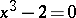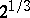# Duplication of the cube

(diff) ← Older revision | Latest revision (diff) | Newer revision → (diff)
Jump to: navigation, search

The problem of constructing a cube having twice the volume of a given cube; it is one of the classical problems of Antiquity, to find an exact construction with ruler and compass. If an edge of the given cube has length 1, the lengthof an edge of the desired cube is equal toand is determined by the cubic equation. However, an exact construction of the segmentby means of ruler and compass is impossible, in view of the unsolvability of the cubic equation by square roots. The first rigorous proof of the unsolvability of the problem of the duplication of the cube by ruler and compass was given in 1837 by P. Wantzell.